Next: Propagator in Relativistic Theory Up: Propagator Methods Previous: Propagator Theory

# The Nonrelativistic Propagator

Our goal is to investigate the differential equation which defines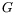, and in particular to solve for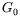explicitly, so that the expansion forcan be explicitly carried out. From Huygens' principle,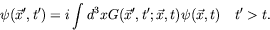(6.28)

A form valid for all time is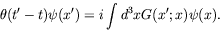(6.29)

Using the chain rule and the fact that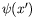satisfies the Schrödinger equation, we have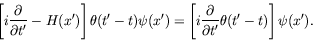(6.30)

Since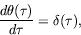(6.31)

we have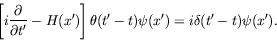(6.32)

Substituting equation 6.29 for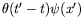, we have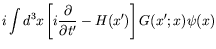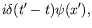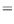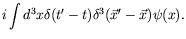(6.33)

Therefore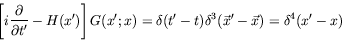(6.34)

is the Green's function equation in the Schrödinger theory. Along with the boundary conditions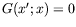for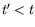this defines the retarded Green's function.

For the free-particle propagator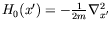.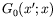can depend only upon the difference of the coordinates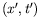and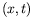. This is because the wave at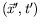emerging from a unit source at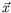which is turned on at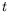depends only on the interval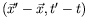, andis precisely the amplitude of this wave.

Consider the Fourier transform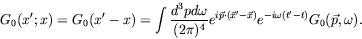(6.35)

The Schrödinger equation for the Green's function becomes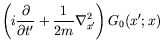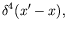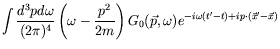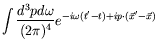(6.36)

and hence for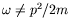,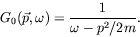(6.37)

To complete the expression, we must have a rule for handling the singularity. We will see that the retarded boundary condition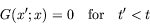(6.38)

requires us to add a positive infinitesimal imaginary part to the denominator. Lets integrate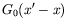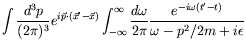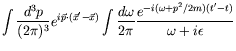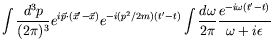(6.39)

The integral over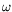is evaluated as a contour integral in the complex-plane as shown in figure 6.3. For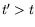, the contour may be closed along an infinite semicircle below the real axis in order to ensure exponential damping of the integrand, and the value of the integral is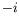by Cauchy's theorem. For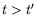, the contour is closed above and the integral vanishes because the pole at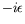lies outside the contour. The integral is thus justtimes the step function. Continuing our previous integration, we have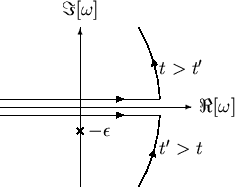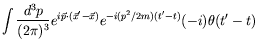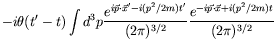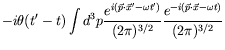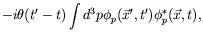(6.40)

where the subscript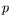stands for plane waves. This is a special case for plane waves. In general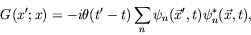(6.41)

where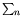is a generalized sum and integral over the spectrum of quantum numbers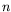,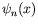is a complete set of normalized solutions to the Schrödinger equation which satisfy a completeness statement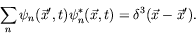(6.42)

There is an enormous amount of information contained in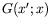. All the solutions of the Schrödinger equation, including the bound states, as required in the completeness relation, appear with equal weight. It is no wonder thatis difficult to compute.

The same Green's function which propagates a solution of the Schrödinger equation forward in time propagates its complex conjugate backward in time. From (6.41) we have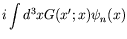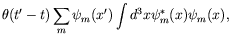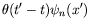(6.43)

and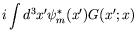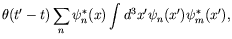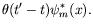(6.44)Next: Propagator in Relativistic Theory Up: Propagator Methods Previous: Propagator Theory
Douglas M. Gingrich (gingrich@ ualberta.ca)
2004-03-18